# Chapter 1 Summary and Review Lets play JEOPARDY

• Slides: 8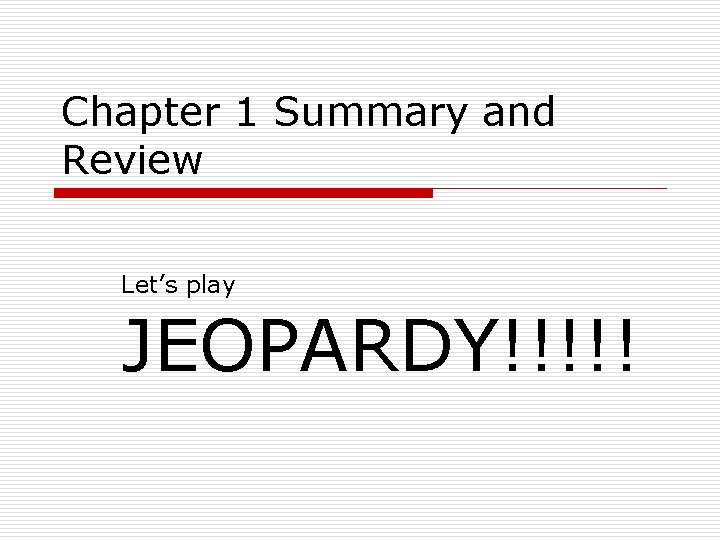Chapter 1 Summary and Review Let’s play JEOPARDY!!!!!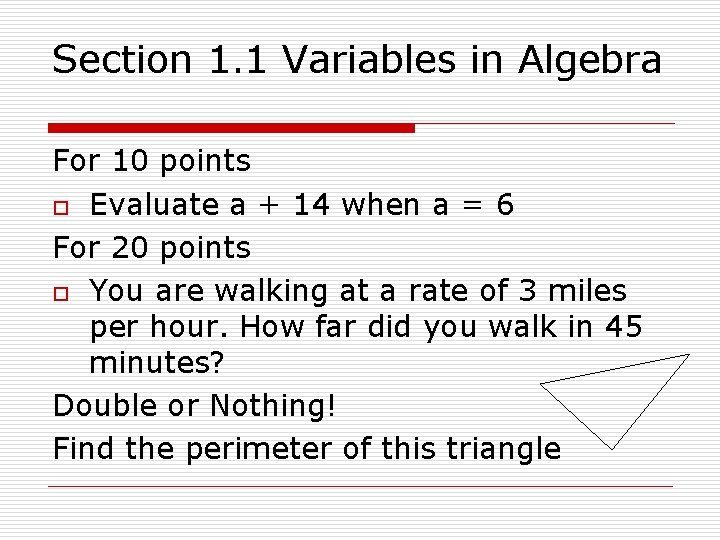Section 1. 1 Variables in Algebra For 10 points o Evaluate a + 14 when a = 6 For 20 points o You are walking at a rate of 3 miles per hour. How far did you walk in 45 minutes? Double or Nothing! Find the perimeter of this triangleSection 1. 2 Exponents and Powers For 10 points Write eight to the fourth power in symbols For 20 points Evaluate (3 x)4 when x = 2 For 30 points Evaluate 6 + (b)3 when b = 6 For Double or Nothing! (3 x)2 + 4 x 3 when x = 3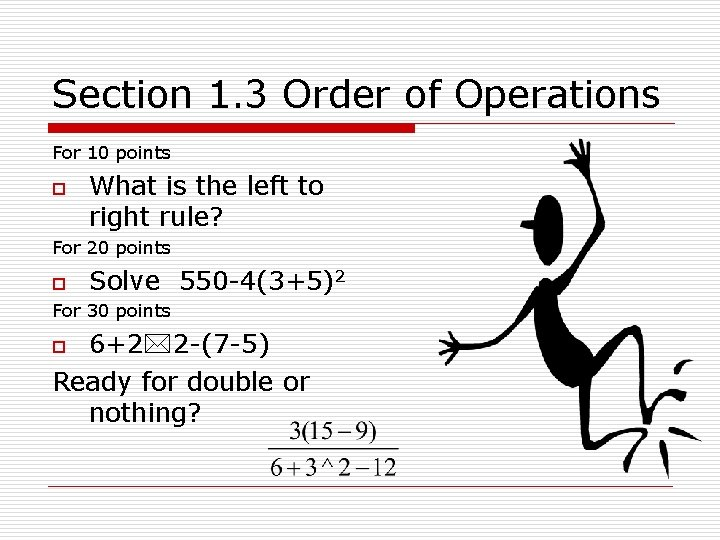Section 1. 3 Order of Operations For 10 points o What is the left to right rule? For 20 points o Solve 550 -4(3+5)2 For 30 points 6+2 2 -(7 -5) Ready for double or nothing? oSection 1. 4 a Equations For 10 points o Is a = 4 a solution to 2 a-3 =2? For 20 points o x 2 – x = 2 Is 3 a solution? For 50 points o The length of a rectangle is 2 x. The width is x. If the perimeter is 30 inches, find the solution for x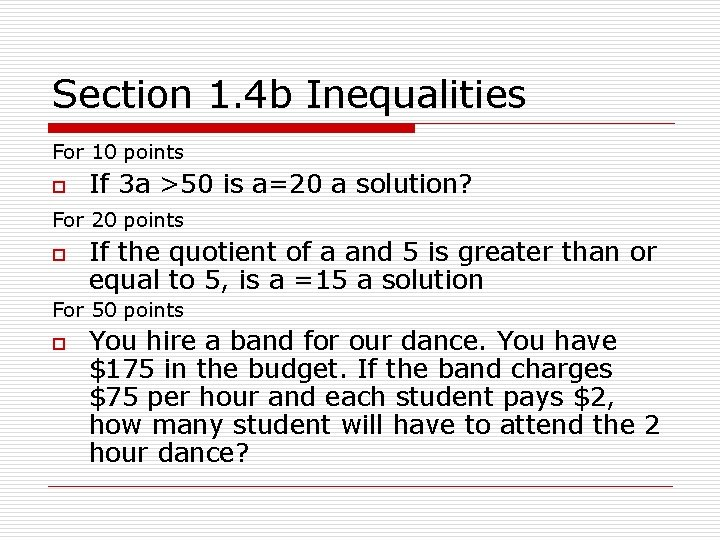Section 1. 4 b Inequalities For 10 points o If 3 a >50 is a=20 a solution? For 20 points o If the quotient of a and 5 is greater than or equal to 5, is a =15 a solution For 50 points o You hire a band for our dance. You have \$175 in the budget. If the band charges \$75 per hour and each student pays \$2, how many student will have to attend the 2 hour dance?Section 1. 5 Translating Symbols For 10 points o Translate the sum of a number and 5 is equal to 15 For 20 points o Translate the difference of x and 7 is less than or equal to 25 For Double your WHOLE score o Seven less than twice a number is less than or equal to 26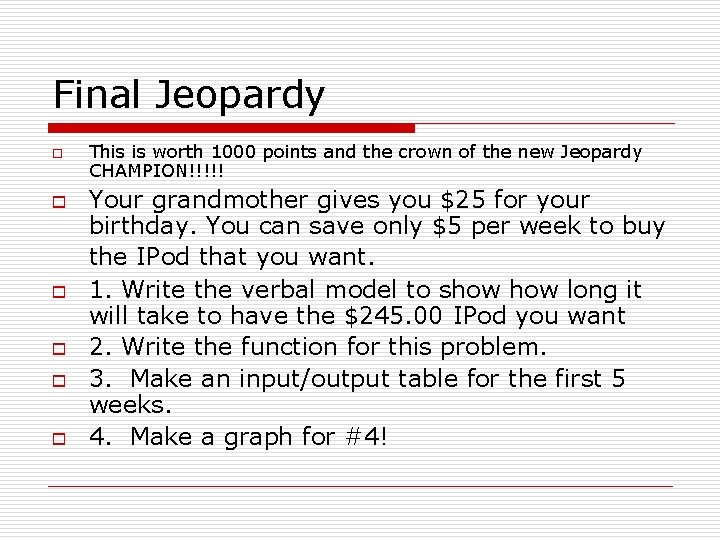Final Jeopardy o o o This is worth 1000 points and the crown of the new Jeopardy CHAMPION!!!!! Your grandmother gives you \$25 for your birthday. You can save only \$5 per week to buy the IPod that you want. 1. Write the verbal model to show long it will take to have the \$245. 00 IPod you want 2. Write the function for this problem. 3. Make an input/output table for the first 5 weeks. 4. Make a graph for #4!